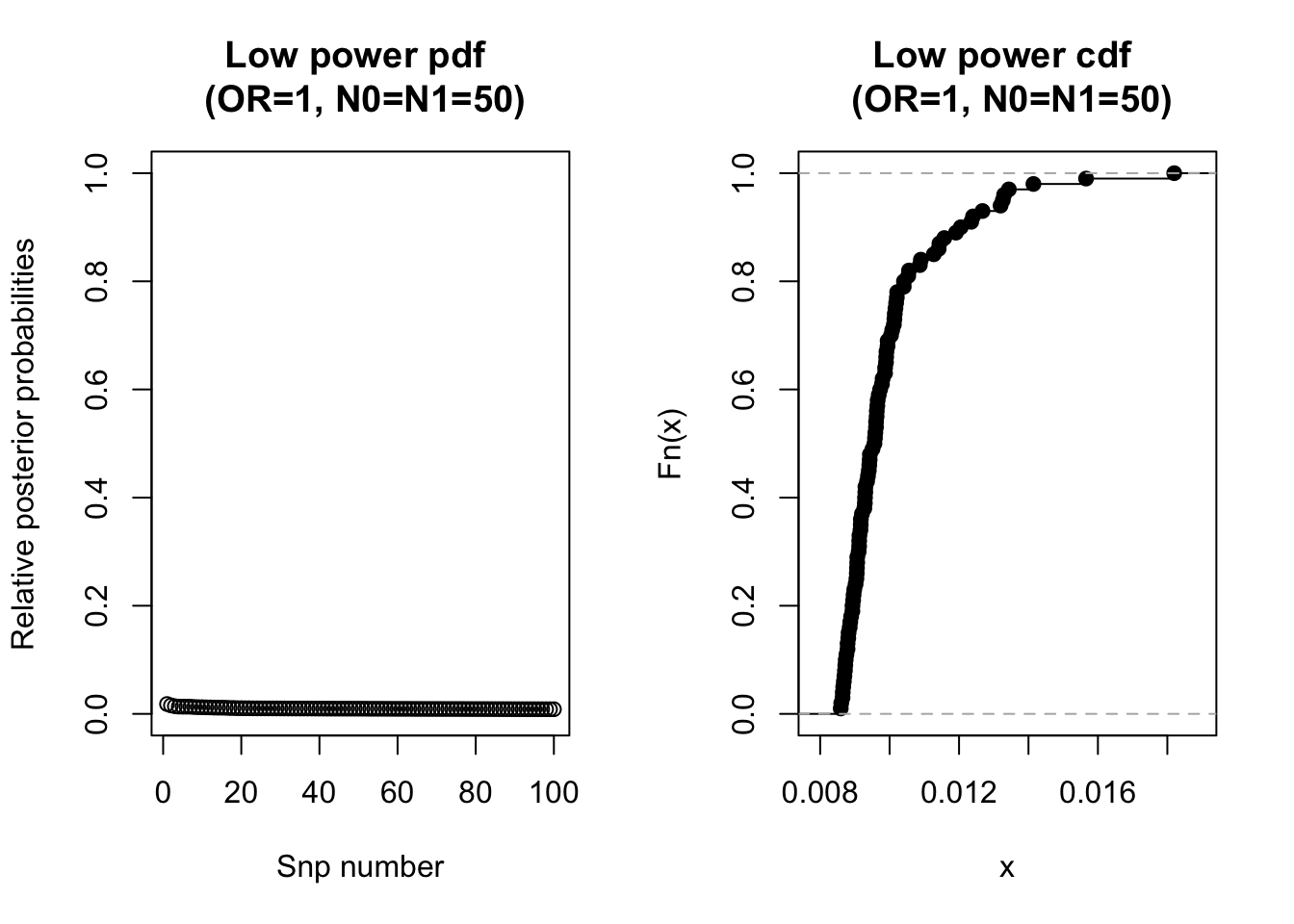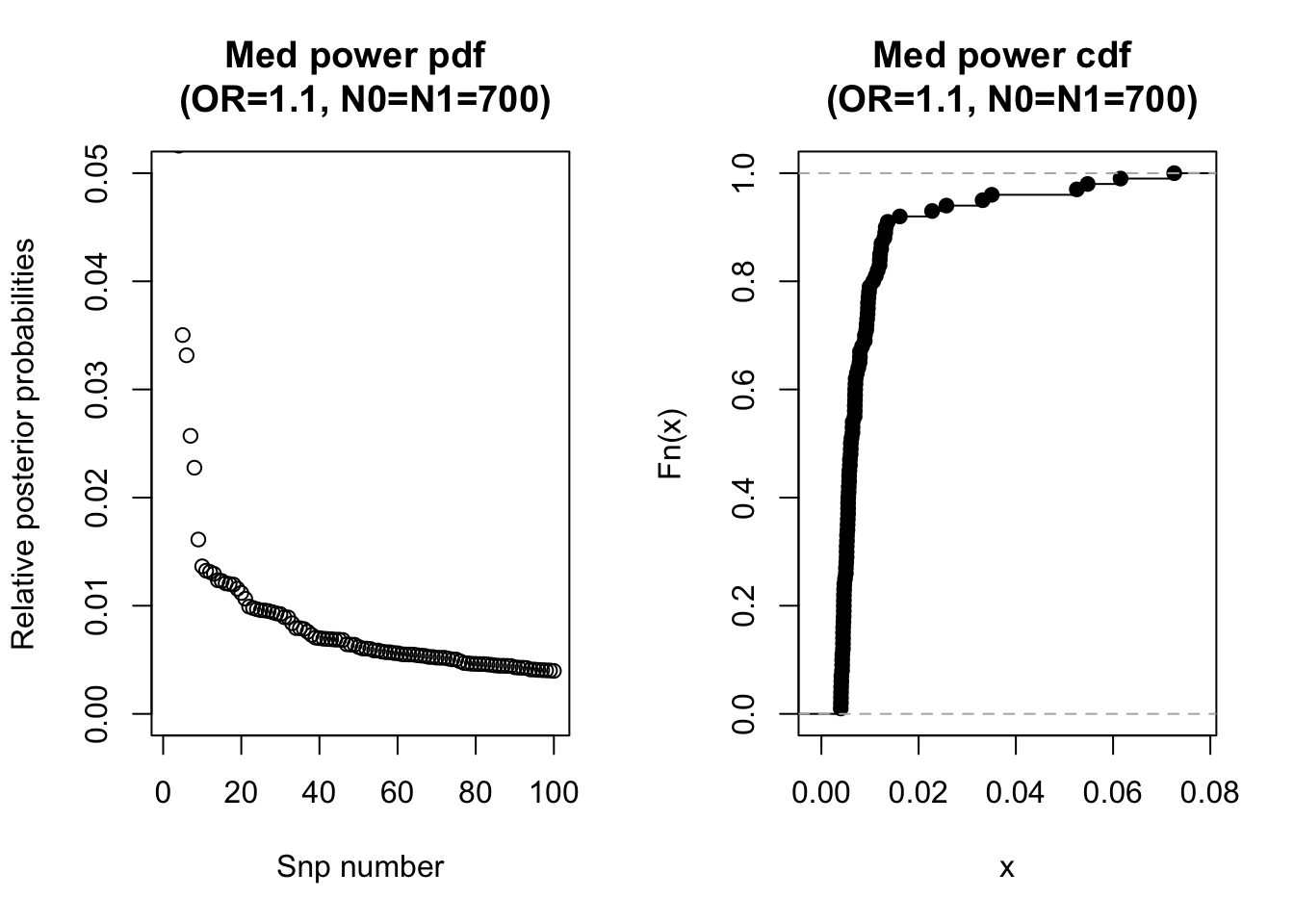### Introduction

A common problem with Bayesian fine-mapping approaches is the large number of variants contained in the credible set. Moreover, we have identified instances of over- and under- coverage, whereby less or more variants respectively are needed in the credible set to accurately reach the $$\alpha$$ credible set size (often $$\alpha=95\%$$). We hope to amend this problem by developing a correction factor, which when applied to the data, will rectify instances of over- and under- coverage.

Schaid et al. (2018) state that the expected posterior probability depends on the effect size of the causal SNP on a trait (OR), the sample size (N), the number of SNPs (nsnps) and SNP correlation structure (LD). Since experimentors know the sample size, the number of SNPs analysed and have some knowledge of the correlation structure, I wish to find a way for experimentors to quantify the information OR provides to the system, without actually having to know the OR. This could then be used in the correction factor to improve coverage estimates in Bayesian fine-mapping experiments.

### Method

Firstly, low, medium and high power systems were constructed to understand the shape of the posterior probability systems and consider ways the OR affects this shape.

Secondly, possible measures of ‘entropy’ are considered. These are measures that I hope quantify the shape of the posterior probability system and the information that the OR provides. These are calculated and plotted for the model systems.

Thirdly, a simulation dataset is created with N, nsnps, thr, MAF and LD fixed. This means that I am considering simulations whereby the variation in the power is only due to variation in the OR. The entropy measures are calculated for each simulated system. Logistic regression and random forest methods are used to analyse the effectiveness of the entropy measures in predicting coverage.

Finally, I simulate posterior probability systems with everything fixed except OR. I look at the distribution of the entropy measures for these simulations.

### 1. Construct model low, medium and high power systems

Model posterior probability systems were produced for low, medium and high power systems.

#### Low power system (OR=1, N0=N1=50)

# x.low <- ref()
# test.low <- simdata_x(x.low, OR=1, N0=50, N1=50) # get 100 systems
# t.low <- test.low[][order(-test.low[]$PP),] setwd("/Users/anna/PhD") t.low <- read.table("t.low") head(t.low) ## snp pvalues MAF PP CV ## s76 SNP.76 0.02214234 0.251 0.01819772 FALSE ## s14 SNP.14 0.04174950 0.249 0.01565847 FALSE ## s52 SNP.52 0.03708935 0.133 0.01414266 FALSE ## s17 SNP.17 0.03914875 0.106 0.01343483 FALSE ## s21 SNP.21 0.09245567 0.295 0.01329824 FALSE ## s15 SNP.15 0.08572478 0.240 0.01326113 FALSE The plots below show the pdf and the cdf of the posterior probabilities for this low power system.#### Medium power system (OR=1.1, N0=N1=700) # test.med <- simdata_x(x.low, OR=1.1, N0=700, N1=700) # get 100 systems # t.med <- test.med[][order(-test.med[]$PP),]

setwd("/Users/anna/PhD")
head(t.med)
##        snp     pvalues   MAF         PP    CV
## s13 SNP.13 0.007335678 0.090 0.07256723 FALSE
## s39 SNP.39 0.011852949 0.296 0.06153209 FALSE
## s92 SNP.92 0.013167979 0.133 0.05476122 FALSE
## s99 SNP.99 0.014588567 0.307 0.05254165 FALSE
## s87 SNP.87 0.025801453 0.212 0.03503086 FALSE
## s4   SNP.4 0.019619915 0.049 0.03316034 FALSE

The plots below show the pdf and the cdf of the posterior probabilities for this medium power system.#### High power system (OR=1.3, N0=N1=1000)

# test.high <- simdata_x(x.low, OR=1.3, N0=1000, N1=1000) # get 100 systems
# t.high <- test.high[][order(-test.high[]\$PP),]

setwd("/Users/anna/PhD")
head(t.high)
##        snp      pvalues   MAF           PP    CV
## s64 SNP.64 4.918353e-02 0.089 0.0006014134 FALSE